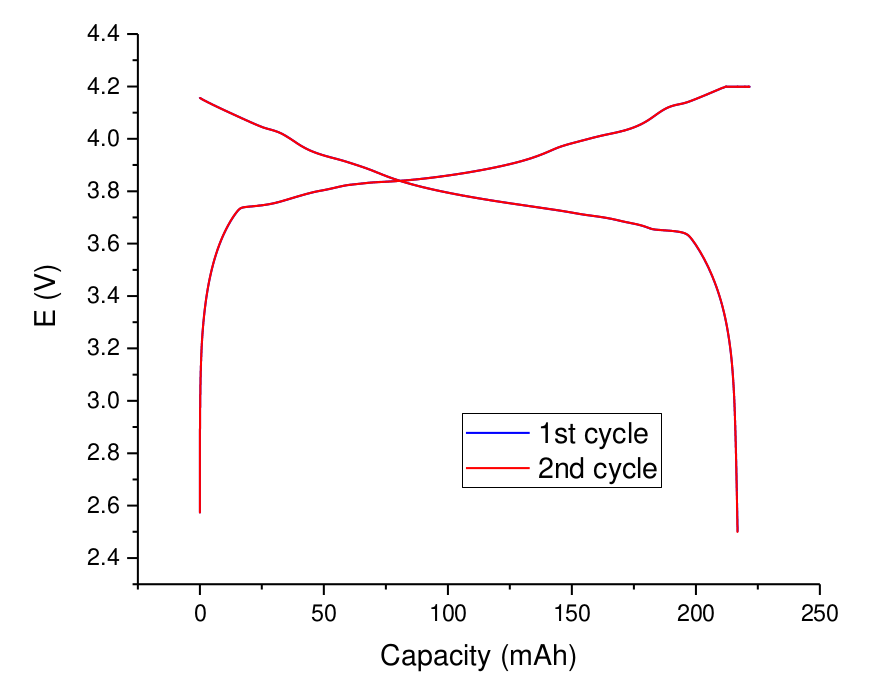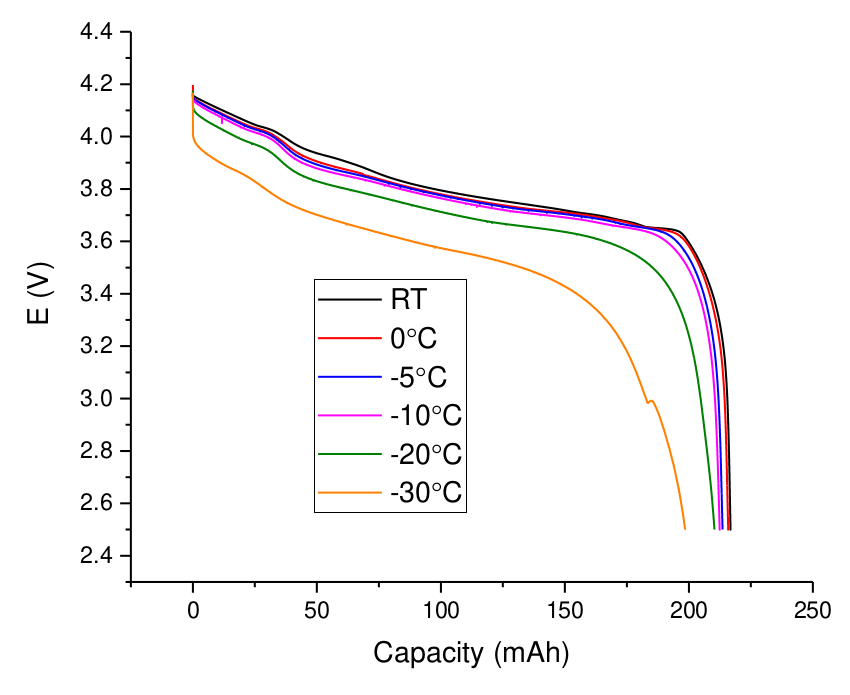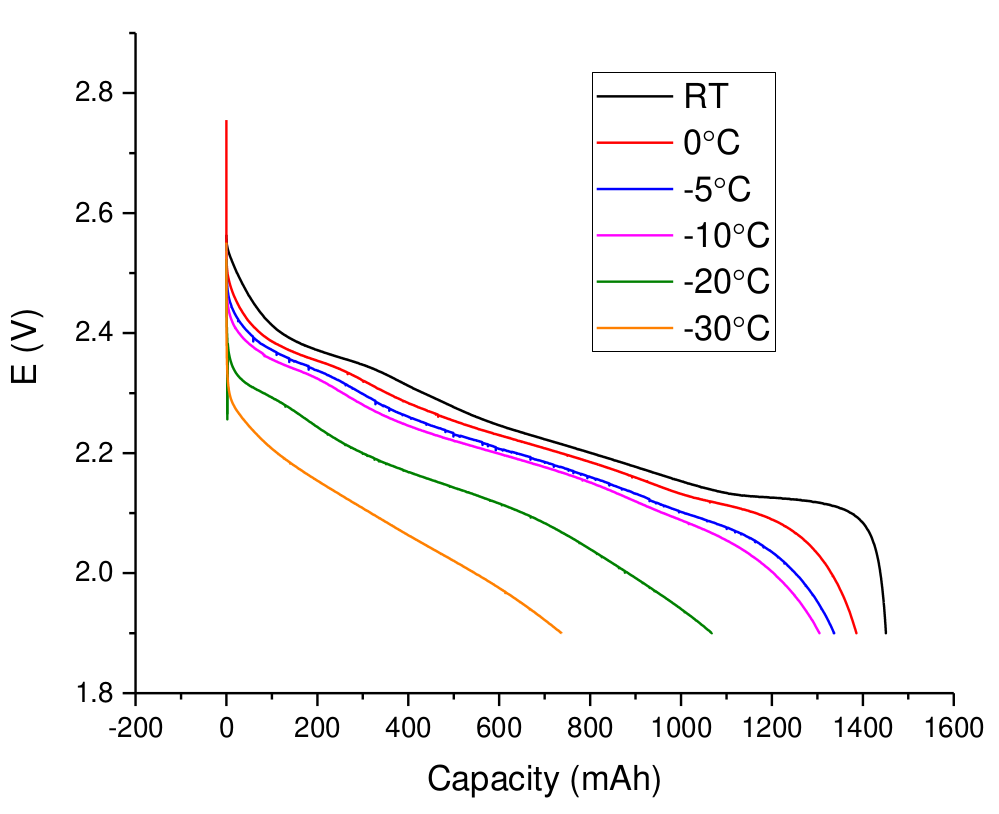https://physics.stackexchange.com/questions/122570/what-is-more-fundamental-fields-or-particles

Numba translates Python functions to optimized machine code at runtime using the industry-standard LLVM compiler library. Numba-compiled numerical algorithms in Python can approach the speeds of C or FORTRAN.

You don't need to replace the Python interpreter, run a separate compilation step, or even have a C/C++ compiler installed. Just apply one of the Numba decorators to your Python function, and Numba does the rest.

``````from numba import jit
import random

@jit(nopython=True)
def monte_carlo_pi(nsamples):
acc = 0
for i in range(nsamples):
x = random.random()
y = random.random()
if (x ** 2 + y ** 2) < 1.0:
acc += 1
return 4.0 * acc / nsamples``````Figure 1. Battery view.

Table 1. Declared characteristics.

 Capacity 220 mAh Nom. voltage 3.7 V Min. voltage 3.0 V Max. voltage 4.2 V Resistance ? mOhm Mass 7.5 g Connector JST-PHR 2.0 plugFigure 2. Galvanostatic charge-discharge curves (22 mA) at room temperature.Figure 3. Galvanostatic charge-discharge curves (22 mA) at different temperatures. Charge is performed at room temperature.Figure 1. LTO battery from China

Table 1. Declared characteristics.

 Capacity 1500 mAh Nom. voltage 2.4 V Min. voltage 1.9 V Max. voltage 2.8 V Resistance 20 mOhm Mass 39 g

# Measured characteristicsFigure 1. Galvanostatic charge-discharge curves depending on current rate. At 2C polarization is ~0.2V, corresponding to ~70 mOhm. The capacity at 2C is less than 1400 mAh.Figure 2. Galvanostatic discharge (150 mA) curves at different temperatures.Figure 3. Cycling at 1 C (1400 mA) charge/discharge.